# Eq2 2

Solve following equation with quadratic members and rational function:

(x2+1)/(x-4) + (x2-1)/(x+3) = 23

Result

x1 =  -2.733
x2 =  5.786
x3 =  8.947

#### Solution:

$(x^2+1)/(x-4) + (x^2-1)/(x+3) = 23 \ \\ x<>4, x<>-3 \ \\ \ \\ (x^2+1) \cdot \ (x+3) + (x^2-1) \cdot \ (x-4) = 23 \cdot \ (x-4) \cdot \ (x+3) \ \\ \ \\ 2 \ x^3 - 24 \ x^2 + 23 \ x + 283 \ \\ \ \\ x_{ 1 } = -2.7333 = -2.733$
$x_{ 2 } = 5.7863 = 5.786$
$x_{ 3 } = 8.9470 = 8.947$

Our examples were largely sent or created by pupils and students themselves. Therefore, we would be pleased if you could send us any errors you found, spelling mistakes, or rephasing the example. Thank you!

Leave us a comment of this math problem and its solution (i.e. if it is still somewhat unclear...):

Showing 1 comment:#### Following knowledge from mathematics are needed to solve this word math problem:

Looking for help with calculating roots of a quadratic equation? Do you have a linear equation or system of equations and looking for its solution? Or do you have quadratic equation?

## Next similar math problems:

1. Right triangle from axesA line segment has its ends on the coordinate axes and forms with them a triangle of area equal to 36 square units. The segment passes through the point ( 5,2). What is the slope of the line segment?
2. Right angled triangle 2LMN is a right angled triangle with vertices at L(1,3), M(3,5) and N(6,n). Given angle LMN is 90° find n
3. Find the 5Find the equation with center at (1,20) which touches the line 8x+5y-19=0
4. Three points 2The three points A(3, 8), B(6, 2) and C(10, 2). The point D is such that the line DA is perpendicular to AB and DC is parallel to AB. Calculate the coordinates of D.
5. LineStraight line passing through points A [-3; 22] and B [33; -2]. Determine the total number of points of the line which both coordinates are positive integers.
6. Center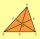In the triangle ABC is point D[1,-2,6], which is the center of the |BC| and point G[8,1,-3], which is the center of gravity of the triangle. Find the coordinates of the vertex A[x,y,z].
7. Slope formFind the equation of a line given the point X(8, 1) and slope -2.8. Arrange your answer in the form y = ax + b, where a, b are the constants.
8. CuboidsTwo separate cuboids with different orientation in space. Determine the angle between them, knowing the direction cosine matrix for each separate cuboid. u1=(0.62955056, 0.094432584, 0.77119944) u2=(0.14484653, 0.9208101, 0.36211633)
9. Find the 10Find the value of t if 2tx+5y-6=0 and 5x-4y+8=0 are perpendicular, parallel, what angle does each of the lines make with the x-axis, find the angle between the lines?
10. Cone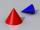If the segment of the line y = -3x +4 that lies in quadrant I is rotated about the y-axis, a cone is formed. What is the volume of the cone?
11. Slope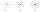Calculate the slope of a line that intersects points (-84,41) and (-76,-32).
12. TriangleTriangle KLM is given by plane coordinates of vertices: K[11, -10] L[10, 12] M[1, 3]. Calculate its area and itsinterior angles.
13. Angle between vectorsFind the angle between the given vectors to the nearest tenth of a degree. u = (-22, 11) and v = (16, 20)
14. Perpendicular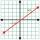What is the slope of the perpendicular bisector of line segment AB if A[9,9] and B[9,-2]?
15. LineLine p passing through A[-10, 6] and has direction vector v=(3, 2). Is point B[7, 30] on the line p?
16. Two peopleTwo straight lines cross at right angles. Two people start simultaneously at the point of intersection. John walking at the rate of 4 kph in one road, Jenelyn walking at the rate of 8 kph on the other road. How long will it take for them to be 20√5 km apar
17. Points collinear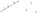Show that the point A(-1,3), B(3,2), C(11,0) are col-linear.